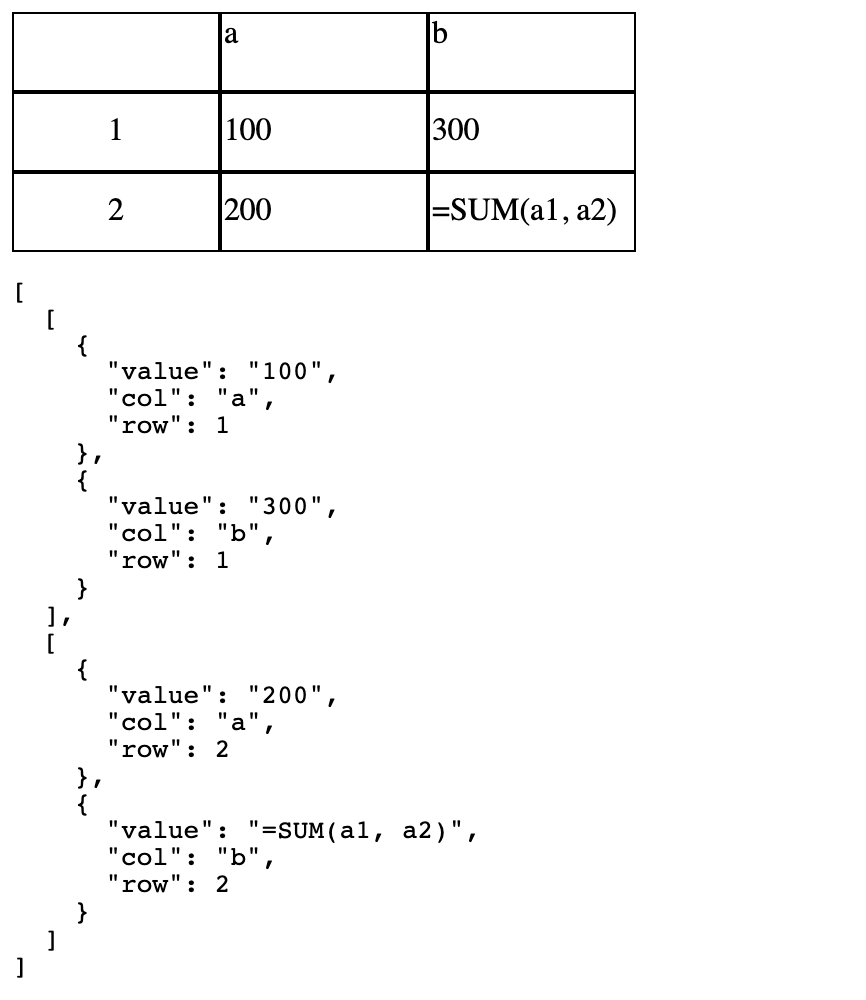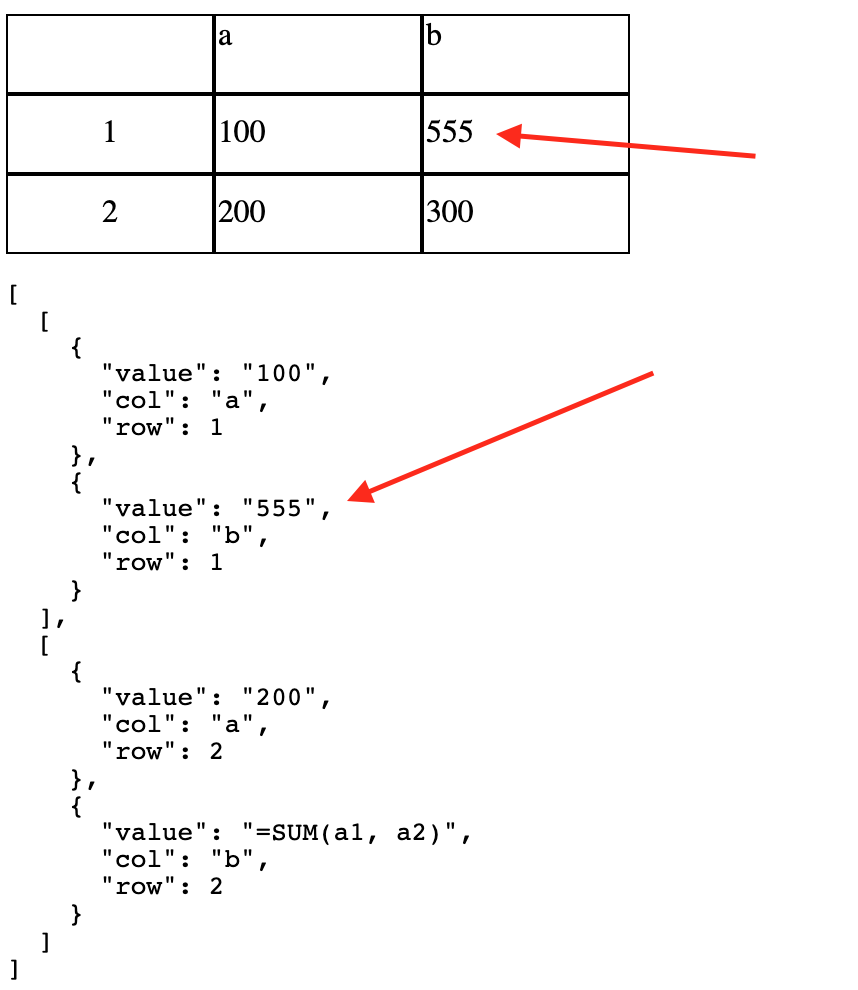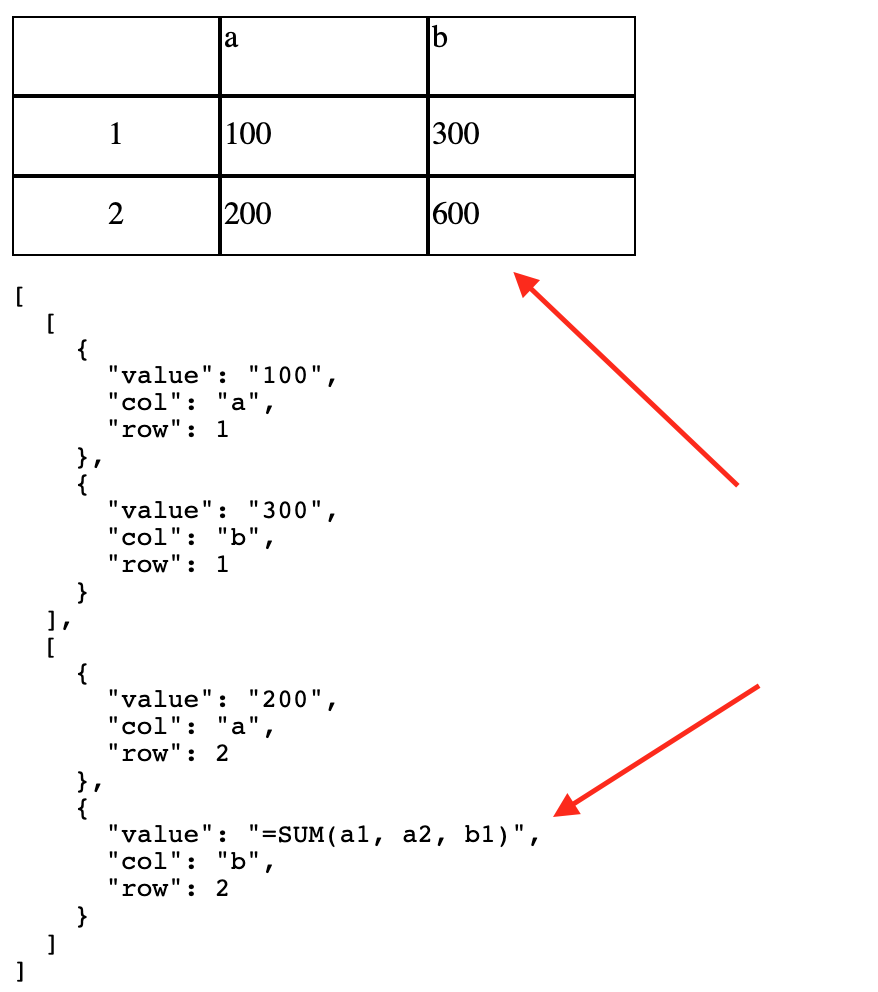# Lazily Evaluated Formulas and useSheet composable

In the previous post, we build a nice little UI layer on top of our spreadsheet engine. The UI is currently read only - our goal will be to allow the user to update cells now, as well as implement basic support for formulas (such as `=SUM(a1, b1)`). We will create a `useSheet` composable, and explore some optimizations using lazily evaluated functions.

You can find the completed source code here.

We left off with our `spreadsheet-editable-cell.vue` looking something like this:

``````<template>
<td v-show="active">
<input :value="cell.value" ref="cellRef" />
</td>

<td
v-show="!active"
@click="activate"
>
<span>
{{ cell.value }}
</span>
</td>
</template>``````

We are not using `v-model`, but `:value` - it’s read only. We need a way to update the cell. If you take a look at any spreadsheet program, you will realize it’s a modal editor, like Vim. The default mode is “visual” - you can see the cells, but typing on your keyboard won’t change them. You need to click a cell to enter “insert” mode - we’ve implemented that. For now, the way I’ve decided to “commit” a change is to update our reactive sheet data structure when the users presses enter. Let’s add an event listener:

``````<template>
<td v-show="active">
<input :value="cell.value" ref="cellRef" @keyup.enter="handleUpdate" />
</td>

<td
v-show="!active"
@click="activate"
>
<span>
{{ cell.value }}
</span>
</td>
</template>``````

Great. Now to figure out how to update the spreadsheet.

## Creating updateCell

At the moment if we want to update the spreadsheet, we will need to `\$emit` an event to the top level component (`app.vue`). This will get out of hand very quickly. Also, updating the spreadsheet is part of the business logic, or in this case, the spreadsheet engine, so we want to extract that logic out of the components as much as possible.

First, let’s add an `updateCell` function to the engine in `spreadsheet.ts`:

``````interface UpdateCell {
index: string
value: string
}

export function updateCell(sheet: Sheet, updateCell: UpdateCell) {
const { index, value } = updateCell
sheet.cells[index].value = value
}``````

Simple stuff - we just pass a `sheet` we’d like to update, and a `cell` with the `index` (eg, `a1` etc) and the new `value`. If you enjoy functional programming, you are probably not too impressed - we are mutating the variable we receive in the arguments, instead of returning a new spreadsheet. Vue’s reactivity relies on mutation, so this is not really avoidable.

Updating the cell is an `O(1)` operation because of our intelligent decision to use a key/value map for storing the spreadsheet. Great. No matter how large the sheet is, it will be quick to update cells.

## Composing with useSheet

We will now write a `useSheet` function to make it easy to access and update the spreadsheet. These functions are sometimes known as “composables”. I created a new directory call `composables` and inside it added a `spreadsheet.ts` file with the following:

``````import { reactive } from 'vue'
import { Sheet  } from '../spreadsheet'

const sheet: Sheet = reactive<Sheet>({
cells: {
'a1': {
value: '100',
type: 'primitive'
},
'a2': {
value: '200',
type: 'primitive'
},
'b1': {
value: '300',
type: 'primitive'
},
'b2': {
value: '=SUM(a1, a2)',
type: 'formula'
},
}
})

export function useSheet() {
return {
sheet
}
} ``````

I added a new cell, `b2`, with a formula for some testing. Other than that, we just moved our `sheet` variable into a new file and exposed it via `useSheet`.

Update `app.vue` to use the new `useSheet` composable:

``````<script lang="ts">
import { computed, reactive } from 'vue'
import { Sheet, render } from './spreadsheet'
import { useSheet } from './composables/spreadsheet'

export default {
components: {
},

setup() {
const { sheet } = useSheet()

return {
sheet: computed(() => render(sheet))
}
}
}
</script>``````

It looks like this:Before going any further, let’s head back to `spreadsheet-editable-cell.vue` and implement `handleUpdate`:

``````// in <script>
import { UICell, updateCell } from './spreadsheet'
import { useSheet } from './composables/spreadsheet'

// ... props ...

setup(props, { emit }) {
const cellRef = ref(null)

const handleUpdate = (\$evt: any) => {
updateCell(
useSheet().sheet,
{
index: `\${props.cell.col}\${props.cell.row}`,
value: \$evt.target.value
}
)
}

const active = computed(() => {
return props.activeCell === `\${props.cell.col}\${props.cell.row}`
})

const activate = () => {
emit('activate', {
cell: props.cell,
cellRef
})
}

return {
active,
cellRef,
handleUpdate,
activate
}
}``````

It works! If you change the value of a cell and press enter, you can see the debugging information update.You may have noticed we are showing `=SUM(a1, a2)` in `b2`. That’s not right. This highlights a problem - to support formulas, we need both a value and a displayValue. The value for the formula would be `=SUM(a1, a2)` and the displayValue would be the resulting calculation.

## Supporting Lazily Evaluated Formulas

Let’s add a new property to the cells: `displayValue`. This will be lazily evaluated. What this means if we won’t calculate the value until we need to. For example, imagine you had 5000 rows. You would only want to calculate the derived values for the cells on screen right now - this is a major optimization, especially if you have a number of complex formulas. We will implement `displayValue` as a function that will return the `value` for `primitive` cells and perform the calculation for `formula` cells.

Let’s start with a test for a function called `deriveValue`. This will parse the formula and calculate the final result. Update `spreadsheet.spec.ts`:

``````import { Dimensions, calcMaxDimensions, Cell, Sheet, render, UICell, deriveValue } from './spreadsheet'

const sheet: Sheet = {
cells: {
'a1': {
value: '100',
type: 'primitive'
},
'a2': {
value: '200',
type: 'primitive'
},
'b1': {
value: '300',
type: 'primitive'
},
'b2': {
value: '=SUM(a1, a2)',
type: 'formula'
},
}
}

test('deriveValue', () => {
const actual = deriveValue(sheet, sheet.cells['b2'])
console.log(actual)

expect(actual).toBe('300')
})``````

Next add a new property to `UICell`. Notice we are using `UICell` - the derived result for a formula is a UI concern. Until someone actually looks at the spreadsheet, we don’t really care what the resulting calculation is, so there is no need to add this information to the internal `Cell` interface.

``````export interface UICell {
value: string
displayValue: () => string
col: string // a, b, c.... z
row: number // 1 2 3....
}``````

Next update `render` to populate the `displayValue` property:

``````export function render(sheet: Sheet): UICell[][] {
const rendered: UICell[][] = []
const { cols, rows } = calcMaxDimensions(sheet)

for (let j = 0; j < rows; j++) {
const row: UICell[] = []

for (let i = 0; i < cols; i++) {
const letter = String.fromCharCode(i + 'a'.charCodeAt(0))
const idx = `\${letter}\${j + 1}`
const cell = sheet.cells[idx]

row.push({
value: cell?.value || '',
col: letter,
displayValue: displayValueFactory(sheet, cell),
row: j + 1
})
}
rendered.push(row)
}

return rendered
}``````

The `render` test is now failing - we added `displayValue`, so we will need to update the test as well. It’s not difficult. For now, though, let’s focus on finishing the `displayValueFactory` function and getting `deriveValue` to pass, then we can fix the test. For now I just commented out the `render` test.

`displayValueFactory` will return a function that calculates the display value. For now, I just want to get it working, so we will assume the formula is going to be a `SUM` formula, and it will always contain a valid formula (eg, any cell we pass it will definitely have an integer). We will add more checks and support for additional formulas later, as well as consider nested formulas, etc.

``````export function displayValueFactory(sheet: Sheet, cell?: Cell) {
if (!cell) {
return () => ''
}

if (cell.type === 'primitive') {
return () => cell.value
}

if (cell.type === 'formula') {
return () => deriveValue(sheet, cell)
}
}``````

For `primitive` we just return the value as is. Finally, let’s implement `deriveValue` and get our test to pass:

``````export function deriveValue(sheet: Sheet, cell: Cell) {
const [_, args] = cell.value.match(/=SUM\((.*)\)/)
const cells = args.split(',').map(x => parseInt(
sheet.cells[x.trim()].value)
)

return cells.reduce((acc, curr) => acc + curr, 0).toString()
}``````

We just grab the arguments to the formula with regexp, assume it’s a SUM formula, assume the arguments are valid cells with valid integers and add them up. Perfect! Of course in a real world system (and even this small one) we need some defensive checks for bad data - we will add those later on.

## Using displayValue

Update `spreadsheet-editable-cell.vue` to use the new `displayValue` property:

``````<template>
<td v-show="active">
<input :value="cell.value" ref="cellRef" @keyup.enter="handleUpdate" />
</td>

<td
v-show="!active"
@click="activate"
>
<span>
{{ cell.displayValue() }}
</span>
</td>
</template>``````

It works. I update the formula in `b2` and pressed enter, then clicked away. The cell value changed to 600, which is correct.We made great progress! We can now update the spreadsheet and handle basic formulas. The next step will be to ensure we correctly handle (or gracefully fail) for invalid formulas, and support more (or even arbitrarily complex?) formulas.

You can find the completed source code here.

Get occasional emails about new content and blog posts.
Absolutely no unsolicted spam. Unsubscribe anytime.
Thanks for registering!